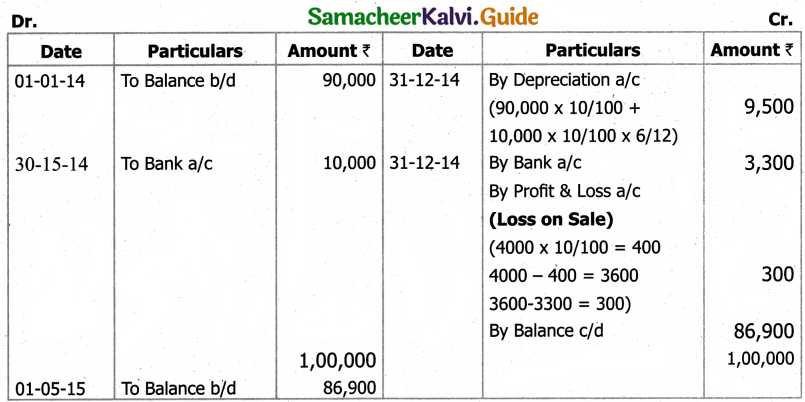Tamilnadu State Board New Syllabus Samacheer Kalvi 11th Accountancy Guide Pdf Chapter 10 Depreciation Accounting Text Book Back Questions and Answers, Notes.

Tamilnadu Samacheer Kalvi 11th Accountancy Solutions Chapter 10 Depreciation Accounting

11th Accountancy Guide Depreciation Accounting Text Book Back Questions and AnswersI. Multiple Choice Questions

Question 1.
Under straight line method, the amount of depreciation is _______.
a) Increasing every year
b) Decreasing every year
c) Constant for all the years
d) Fluctuating every year
c) Constant for all the years

Question 2.
If the total charge of depreciation and maintenance cost are considered, the method that provides a uniform charge is _______.
a) Straight line method
b) Diminishing balance method
c) Annuity method
d) Insurance policy method
b) Diminishing balance method

Question 3.
Under the written down value method of depreciation, the amount of depreciation is _______.
a) Uniform in all the years
b) Decreasing every year
c) Increasing every year
d) None of the above
b) Decreasing every year

Question 4.
Depreciation provided on machinery is debited to _______.
a) Depreciation account
b) Machinery account
d) Provision for depreciation account
a) Depreciation accountQuestion 5.
Cash received from sale of fixed asset is credited to _______.
a) Profit and loss account
b) Fixed asset account
c) Depreciation account
d) Bank account
b) Fixed asset account

Question 6.
Depreciation is provided on _______.
a) Fixed assets
b) Current assets
c) Outstanding charges
d) All assets
a) Fixed assets

Question 7.
Depreciation is caused by _______.
a) Lapse of time
b) Usage
c) Obsolescence
d) a, b and c
d) a, b and c

Question 8.
Depreciation is the process of _______.
a) Allocation of cost of the asset to the period of its useful life
b) Valuation of assets
c) Maintenance of an asset in a state of efficiency
d) Adding value to the asset
a) Allocation of cost of the asset to the period of its useful life

Question 9.
For which of the following assets, the depletion method is adopted for writing off cost of the asset?
a) Plant and machinery
b) Mines and quarries
c) Buildings
b) Mines and quarries

Question 10.
A depreciable asset may suffer obsolescence due to _______.
a) Passage of time
b) Wear and tear
c) Technological changes
d) None of the above.
c) Technological changes

Question 11.
Which method shall be efficient, if repairs and maintenance cost of an asset increases as it grows older.
a) Straight line method
b) Reducing balance method
c) Sinking fund method
d) Annuity method
b) Reducing balance methodQuestion 12.
Depreciation is to be calculated from the date when _______.
a) Asset is put to use
d) Invoice of assets is received
a) Asset is put to use

Question 13.
If the rate of depreciation is same, then the amount of depreciation under straight line method vis-a-written down value method will be _______.
a) Equal in all years
b) Equal in the first year but higher in subsequent years
c) Equal in the first year but lower in subsequent years
d) Lower in the first year but equal in subsequent years.
b) Equal in the first year but higher in subsequent years

Question 14.
Residual value of an asset means the amount that it can fetch on sale at the _______ of its useful life.
a) Beginning
b) End
c) Middle
d) None
b) EndII. Very Short Answer Type Questions

Question 1.
What is meant by depreciation?

• The process of allocation of the relevant cost of a fixed asset over its useful life is known asdepreciation.
• It is an allocation of cost against the benefit derived from a fixed asset during an accounting period.

Question 2.
List out the various methods of depreciation.
The following are the different methods of providing depreciation

• Straight line method or Fixed installment method or Original cost method
• Written down value method or Diminishing balance method
• Sum of years of digits method
• Machine hour rate method
• Depletion method
• Annuity method.
• Revaluation method
• Sinking fund method
• Insurance policy method

Question 3.
Give the formula to find out the amount and rate of depreciation under straight line method of depreciation.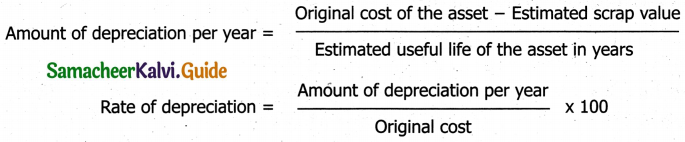Question 4.
What is annuity method?
1. Under this method, not only the original cost of the asset but also the amount of interest on the investment is taken into account while computing depreciation.

2. The idea of considering interest is that if the investment is made in any other asset instead of the relevant fixed asset, it would have earned a certain rate of interest.

3. To calculate the amount of depreciation, annuity factor is used. Annuity factor can be found out from the annuity table or by using formula.

4. Amount of depreciation is computed as follows:
Amount of depreciation = Annuity factor x Original cost of the asset

Question 5.
What is sinking fund method?
1. Sinking fund method is adopted especially when it is desired not merely to write off an asset but also to provide enough funds to replace an asset at the end of its working life.

2. Under this method, theamount charged as depreciation is transferred to depreciation fund and invested outside thebusiness.

3. This method of depreciation is suitable for assets of higher value.

4. This method is also known as depreciation fund method.

5. This method not only takes into account depreciation but also makes provision for the replacement of the asset.Question 1.
What are the objectives of providing depreciation?
Following are the objectives of providing depreciation:

1. To find out the true profit:

• According to matching principle, the expenses incurred during a period must be matched with reve¬nue earned during that period.
• Hence, when an asset is used for generating income for a business, the cost of the asset attributable to the use, i.e., the reduction in the book value of the asset proportionate to the benefit derived from it, should be charged against the revenue.
• This is to be done to find out the true cost of production and profit or loss of the business for every accounting period.

2. To present the true and fair view of financial position:

• When the depreciation is charged on fixed assets, the book value of fixed assets are reduced to that extent and the remaining value is shown in the balance sheet.
• The balance represents the value of benefit that is yet to be derived from them.
• The written down value is the true value of fixed assets which represent cost not yet written off.
• The balance sheet must represent a true and fair view of financial status.
• Hence, fixed assets must be shown at their at written downvalue.

3. To facilitate replacement of fixed assets:

• When the depreciation is debited to profit and loss account, an equal amount is either retained in the business or invested outside the business.
• When the useful life of an asset comes to anend, a new asset can be purchased by using the resources available in the business.

4. To avail tax benefits:

• As per the Indian Income Tax Act, while computing tax on business income, depreciation is deductible from income.
• Hence, depreciation is computed and charged to profit and iossaccount to reduce tax liability. (v)To comply with legal requirements:
• Depreciation is provided on fixed assets to comply with the provisions of law apart from Income Tax Act.
• For example, Section 123(1) of the Indian Companies Act, 2013, requires every company to provide depreciation on fixed assets before declaring dividend to its shareholders.

Question 2.
What are the causes for depreciation?
1. Wear and Tear:

• The physical deterioration of assets due to normal use is called wear and tear.
• The value of the assets decreases proportionately.

2. Efflux of time:
Some kinds of assets become potentially less useful with the passage of time whether used or not.

3. Obsolescence:

• It is a reduction in the value of assets as a result of the availability of updated alternative assets.
This happens due to new inventions and innovations.
• Though the original asset is in a usable condition, it is not preferred by the users and it loses its value
• For example, preference of latest computers by the users.

• In some cases, the use of assets may be stopped due to their inadequacy for the purpose.
• These may become inadequate due to expansion in the capacity of a firm.

5. Lack of maintenance:

• The good maintenance naturally increase the life of the asset.
• When there is lack of maintenance, there is possibility of more depreciation.

6. Abnormal factors:

• Decline in the usefulness of fixed asset may be caused by abnormal factors like damage due to fire accidents, natural calamities etc.

Question 3.
State the advantages and limitations of straight line method or depreciation.
Following are the merits of straight line method of depreciation
(a) Simple and easy to understand – Computation of depreciation under this method is very simple and easy to understand.

(b) Equality of depreciation burden – Equal amount of depreciation is debited to the profit and loss account each year to reduce the burden of depreciation on the profit of each year is equal.

(c) Assets can be completely written off – The book value of an asset can be reduced to zero if there is no scrap value or to be the scrap value at the end of its useful life. The asset account can be completely written off.

(d) Suitable for the assets having fixed working life – This method is appropriate for the fixed assets having certain fixed period of working life. In such cases, the estimation of useful life is easy and in turn in helps in easy determination of rate of depreciation.

Limitations:
(a) Ignores the actual use of the asset – A fixed amount of depreciation is provided on each asset by applying the predetermined rate of depreciation on its original cost. But the actual use of the asset is not considered in computation of depreciation.

(b) Ignores the interest factor – This method does not take into account the loss of interest on the amount invested in the asset.

(c) Total charge on the assets will be more when the asset becomes older – The amount of depreciation and cost of maintenance put together is less in the initial period and goes up year after year. This method did not concentrate on this.

(d) Difficulty in the determination of scrap value – It may be quite difficult to assess the true scrap value of the asset after a long period after the date of its installation.Question 4.
State the advantages and limitations of written down value method of depreciation.
(a) Equal Charge against income

• In the starting period depreciation is high and repaid charges are low.
• When the asset becomes older, the amount of depreciation charged is less but repair charges are high.
• The total burden on profit in respect of depreciation and repairs put together remains almost similar year after year.

(b) Logical Method:

• In the earlier years, when the asset is more productive, high depreciation is charged.
• In the later years when the asset becomes less productive, the depreciation charge is less.

Limitations:
(a) Assets cannot be completely written off – Under this method, the value of an asset even if it becomes obsolete and useless, cannot be reduced to zero and some balance would continue in the asset account.

(b) Ignores the interest factor – This method does not take into account the loss of interest on the amount invested in the asset. The amount would have earned interest, had it been invested outside the business is not considered.

(c) Difficulty in determining the rate of depreciation – Under this method, the rate of providing depreciation cannot be easily determined. The rate is generally kept higher because it takes very long time to write off an asset down to its scrap value.

(d) Ignores the actual use of the asset – Under this method, a fixed rate of depreciation is provided on the written down value of the asset by applying the predetermined rate of depreciation on its original cost. But the actual use of the asset is not considered in the computation of depreciation.

Question 5.
Distinguish between straight line method and written down value method of providing depreciation.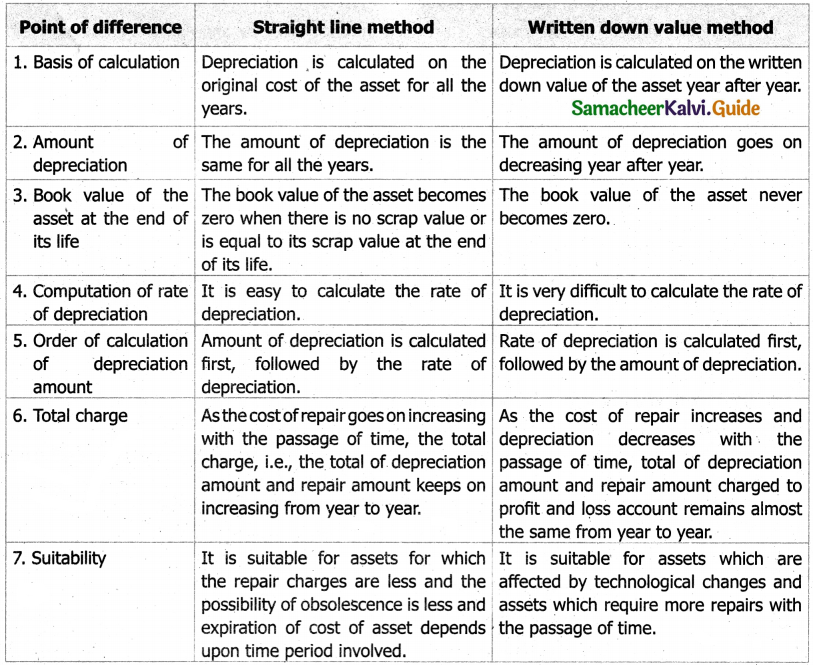IV. Exercises

Straight line Method:

Question 1.
A firm purchased plant for ₹ 40,000. Erection charges amounted to ₹ 2,000. Effective life of the plant is 5 years. Calculate the amount of depreciation per year under straight line method.
Solution: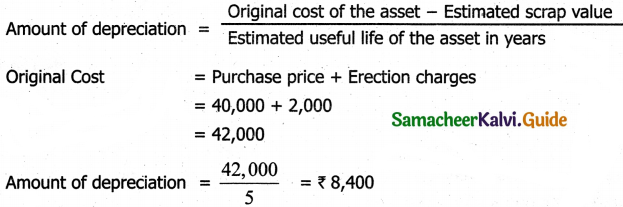Question 2.
A company purchased a building for ₹ 50,000. The useful life of the building is 10 years and the residual value is ₹ 2,000. Find out the amount and rate of depreciation under straight line method.
Solution: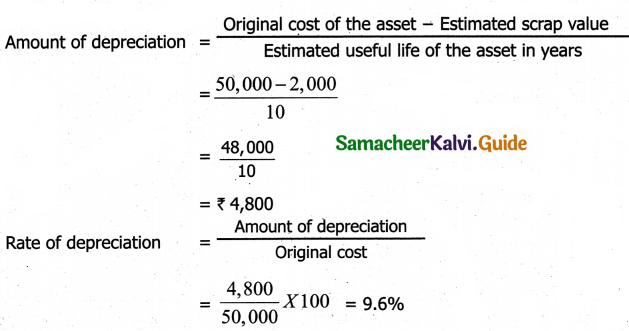Question 3.
Furniture was purchased for ₹ 60,000 on 1-7-2016. It is expected to last for 5 years. Estimated scrap at the end of five years is ₹ 4,000. Find out the rate of depreciation under straight line method.
Solution: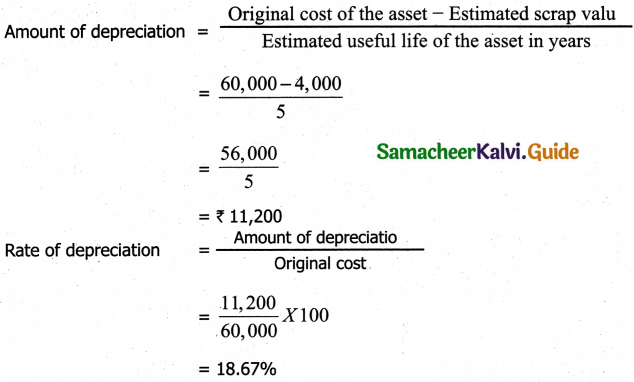Question 4.
Calculate the rate of depreciation under straight line method from the following information
Purchased a second hand machinery on 1.1.2018 for ₹ 38,000
On 1.1.2018 spent ₹ 12,000 on its repairs
Expected useful life of machine is 4 years
Estimated residual value ₹ 6,000
Solution: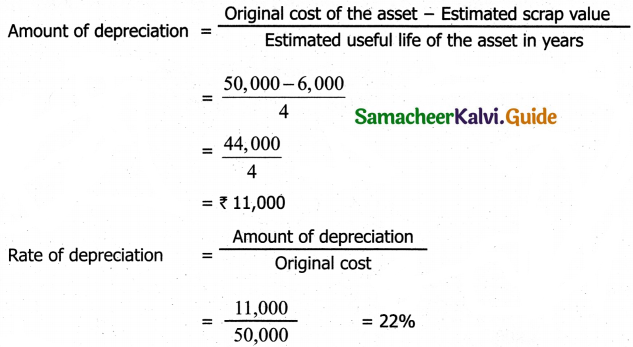Note:
Original cost = Purchases price + repairs = 38,000 + 12,000 = ₹ 50,000

Question 5.
Calculate the rate of depreciation under straight line method.
Purchase price of a machine ₹ 80,000
Expenses to be capitalized ₹ 20,000
Estimated residual value ₹ 4,000
Expected useful life 4 years
Solution: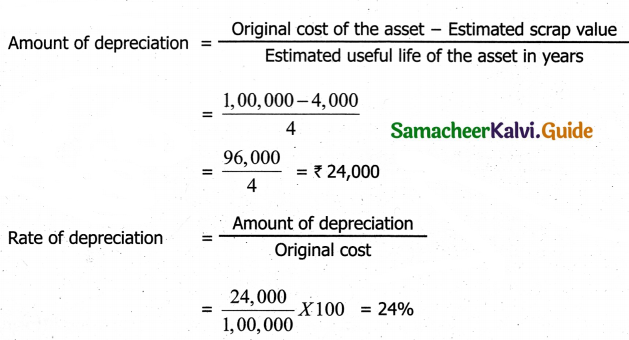Note:
Original cost = Purchases price + Expense to be Capitalized
= 80,000 + 20,000
= ₹ 1,00,000

Question 6.
Machinery was purchased on 1st January 2015 for ₹ 4,00,000. ₹ 15,000 was spent on its erection and ₹ 10,000 on its freight charges. Depreciation is charged at 10% per annum on straight line method. The books are closed on 31st March each year. Calculate the amount of depreciation on machinery for the first two years.
Solution:
Calculation on the amout of depreciation on machinery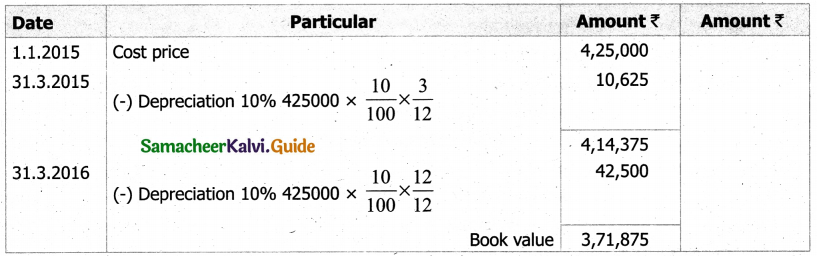Amount of depreciation
31-3-2015 = ₹ 10625
31-3-2016 = ₹ 42500
Note:
Original cost = Purchases price + Erection charges + freight charges
= 4,00,000 + 15,000 + 10,000 = ₹ 4,25,000Question 7.
An asset is purchased on 1.1.2016 for ₹ 25,000. Depreciation is to be provided annually according to straight line method. The useful life of the asset is iO years and its residual value is ₹ 1,000. Accounts are closed on 31st December every year. You are required to find out the rate of depreciation and give journal entries for first two years.
Solution: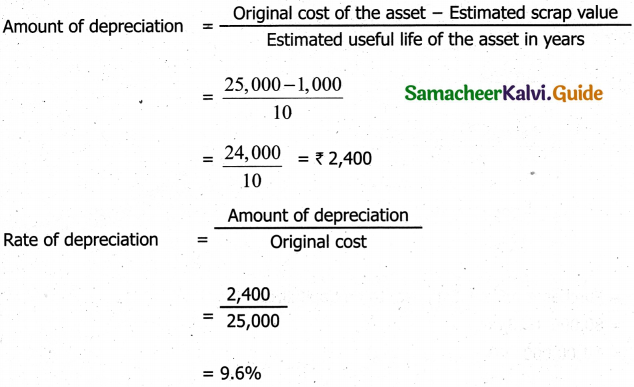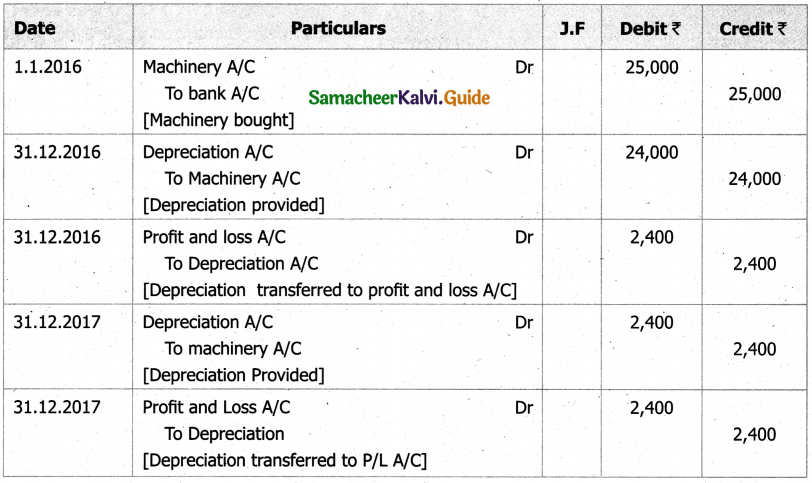Question 8.
From the following particulars, give journal entries for 2 years and prepare machinery account under straight line method of providing depreciation:
Machinery was purchased on 1.1.2016
Price of the machine ₹ 36,000
Freight charges ₹ 2,500
Installation charges ₹ 1,500
Life of the machine 5 years
Solution: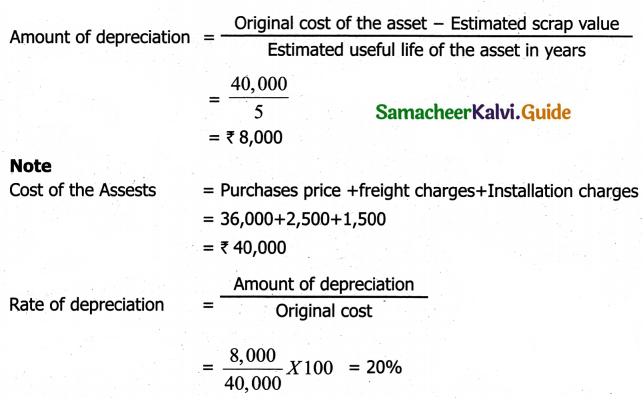Journal entries: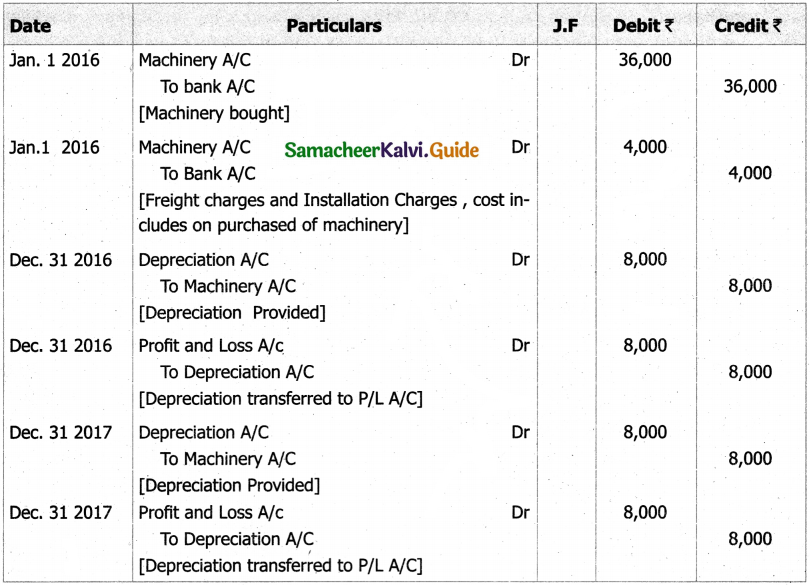Machinary Account: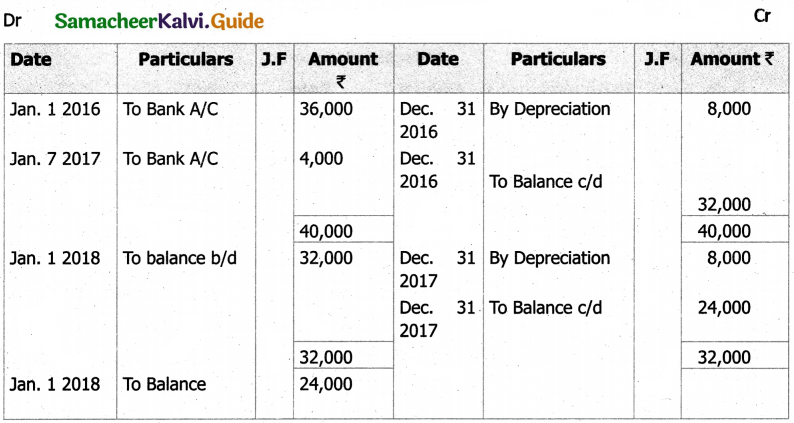Question 9.
A manufacturing company purchased on 1 April, 2010, a plant and machinery for ₹ 4,50,000 and spent ₹ 50,000 on its installation. After having used it for three years, it was sold for ₹ 3,85,000. Depreciation is to be provided every year at the rate of 15% per annum on the fixed installment method. Accounts are closed on 31st March every year.
Solution:
Calculate profit or loss on sale of machinery.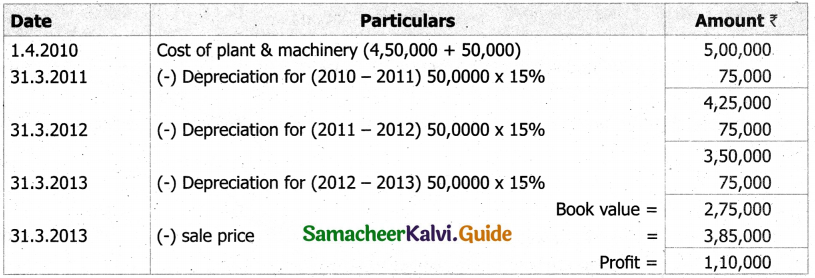Profit on sales = sale price – Book value
= 3,85,000 – 2,75,000
= ₹ 1,10,000

Question 10.
On 1st April 2008, Sudha and Company purchased machinery for ₹ 64,000. To instal the machinery expenses incurred was ₹ 28,000. Depreciate machinery 10% p.a. under straight line method. On 30th June, 2010 the worn out machinery was sold for ₹ 52,000. The books are closed on 31st December every year. Show machinery account.
Solution:
Machinary Account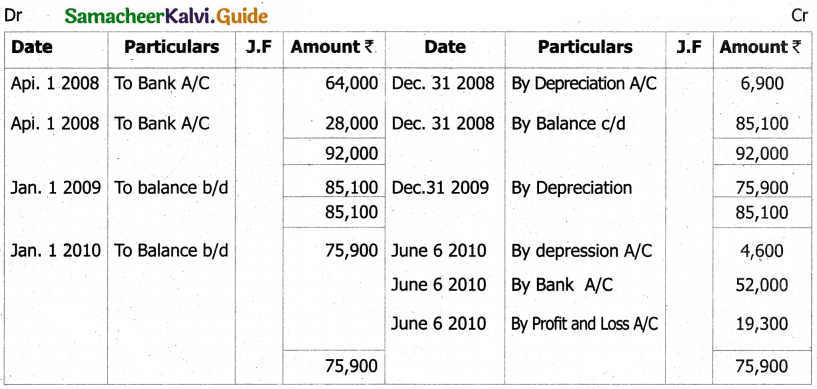Question 11.
Ragul purchased machinery on April 1, 2014 for ₹ 2,00,000. On 1st October 2015, a new machine costing ₹ 1,20,000 was purchased. On 30th September 2016, the machinery purchased on April 1, 2014 was sold for ₹ 1,20,000. Books of accounts are closed on 31st March and depreciation is to be provided at 10% p.a. on straight line method. Prepare machinery account and depreciation account for the years 2014-15 to 2016-17.
Solution:
Machinery Account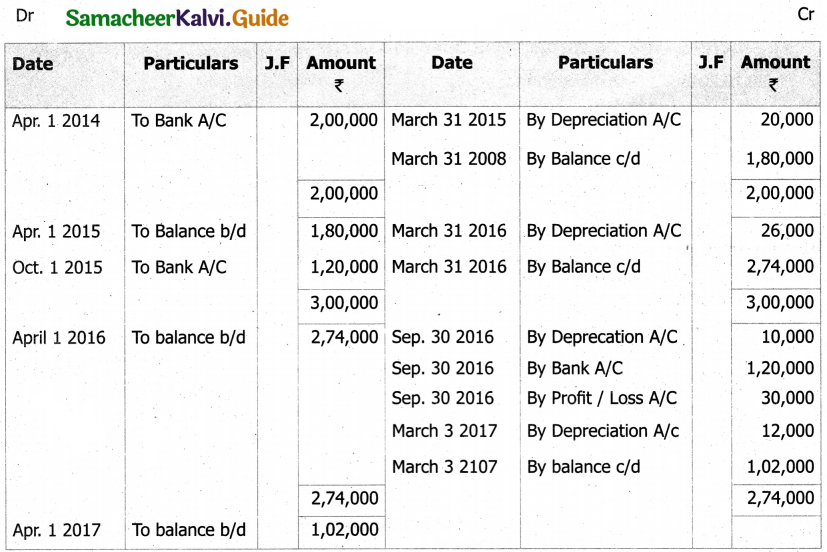Depreciation Account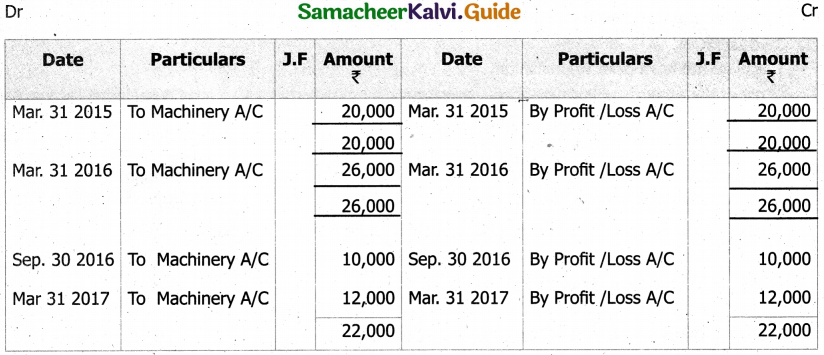Notes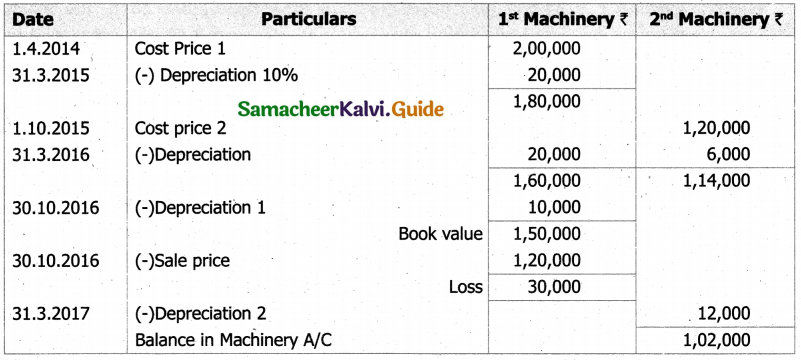Question 12.
An asset is purchased for ₹ 50,000. The rate of depreciation is 15% p.a. Calculate the annual depreciation for the first two years under diminishing balance method.
Solution: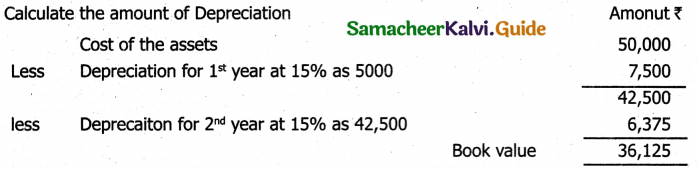Question 13.
A boiler was purchased on 1st January 2015 from abroad for ₹ 10,000. Shipping and forwarding charges amounted to 12,000. Import duty ₹ 7,000 and expenses of installation amounted to ₹ 1,000. Calculate depreciation for the first 3 years @10% p.a. on diminishing balance method assuming that the accounts are dosed 31st December each year.
Solution:
Calculate the amount of Depreciation
Cost of the assets = Purchase price + shipping and forwarding charges + Import duty+Install – action charge.
= 10,000 + 2,000 + 7,000 + 1,000
= ₹ 20,000.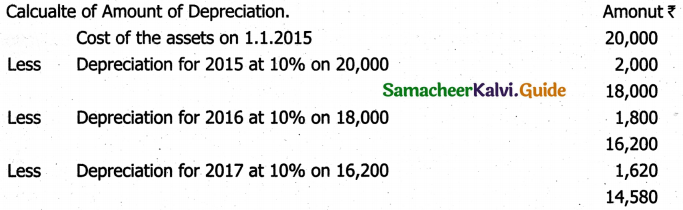Question 14.
A furniture costing ₹ 5,000 was purchased on 1.1.2016, the installation charges being ₹ 1,000. The furniture is to be depreciated @10% p.a. on the diminishing balance method. Pass journal entries for the first two years.
Solution: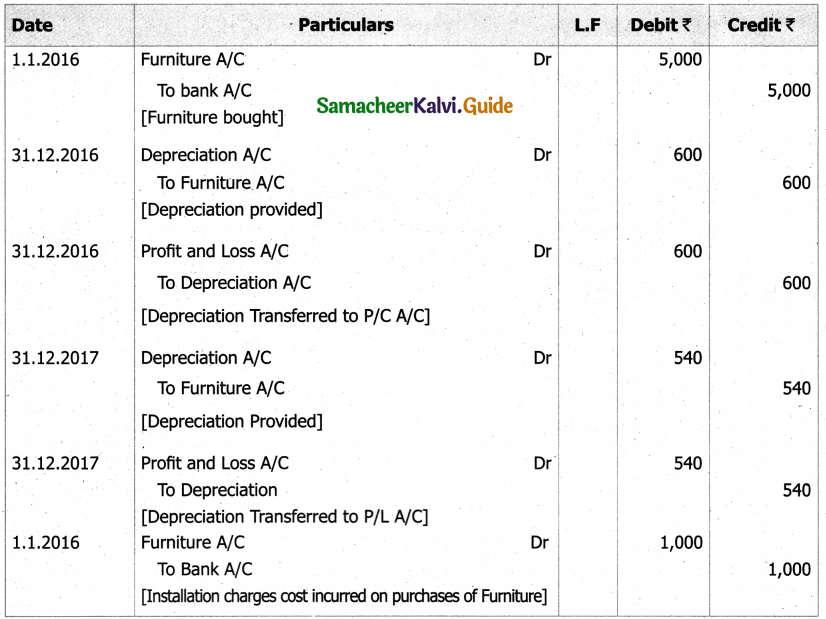Question 15.
A firm acquired a machine on 1st April 2015 at a cost of ₹ 50,000. Its life is 6 years. The firm writes off depreciation @ 30% p.a. on the diminishing balance method. The firm closes its books on 31st December every year. Show the machinery account and depreciation account for three years starting from 1st April 2015.
Solution:
Machinary Account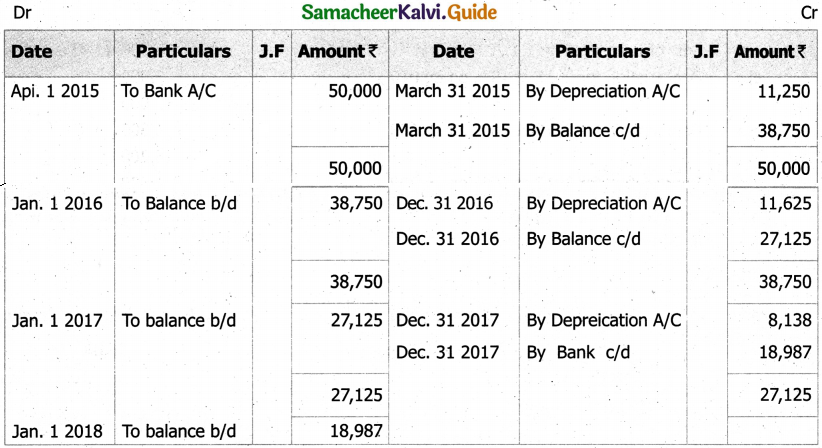Depreciation Account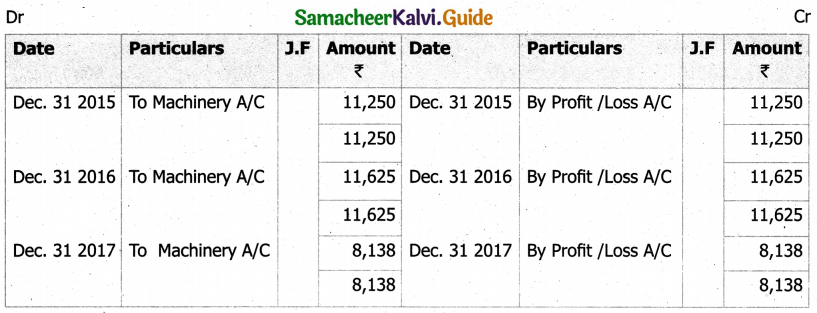Question 16.
A firm purchased a machine for ₹ 1,00,000 on 1-7-2015. Depreciation is written off at 20% on reducing balance method. The firm closes its books on 31st December each year. Show the machinery account upto 31-12-2017.
Solution:
Machinary Account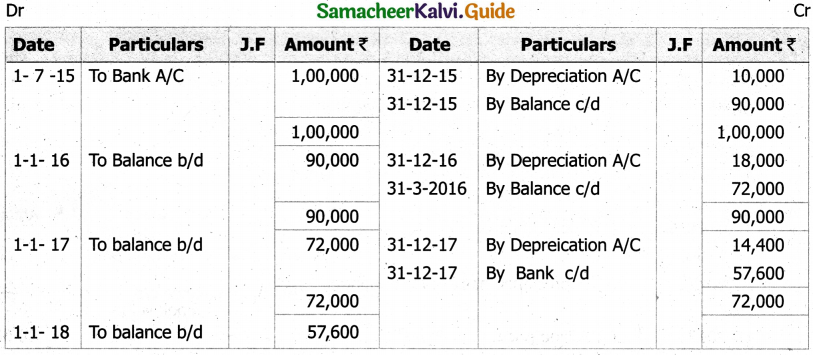Question 17.
On 1st October 2014, a truck was purchased for ₹ 8,00,000 by Laxmi Transports Ltd. Depreciation was provided @ 15% p.a. under diminishing balance method. On 31st March 2017, the above truck was sold for ₹ 5,00,000. Accounts are closed on 31st March every year. Find out the profit or loss made on the sale of the truck.
Solution:
Calculation of Profit (or) Loss on sales of assets.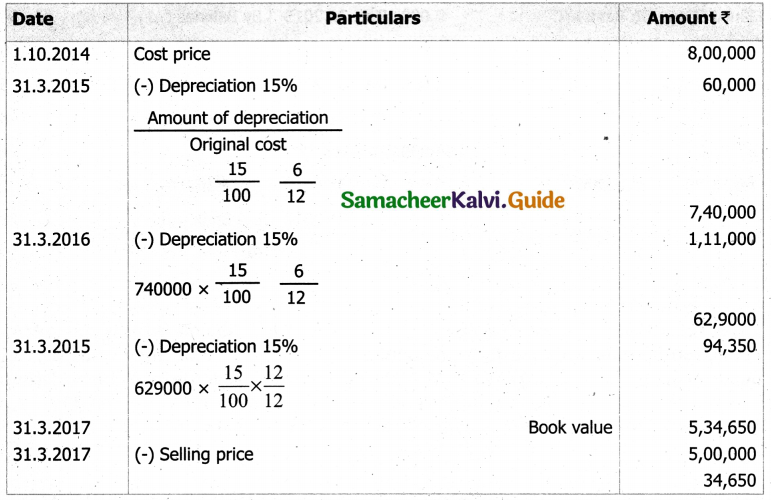Question 18.
On 1st January 2015, a second hand machine was purchased for ₹ 58,000 and ₹ 2,000 was spent on its repairs. On 1st July 2017, it was sold for ₹ 28,600. Prepare the machinery account for the years 2011 to 2013 under written down value method by assuming the rate of depreciation as 10% p.a. and the accounts are dosed on 31st December every year.
Solution: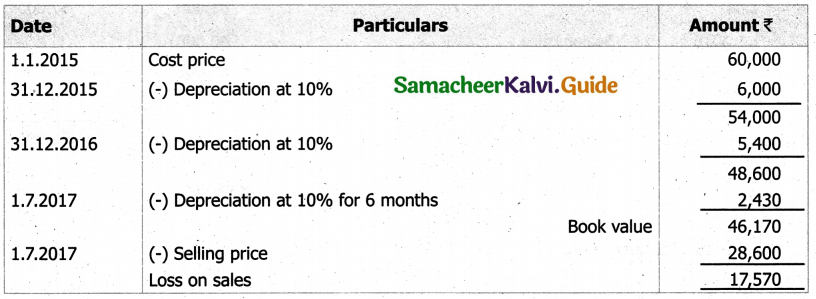Machinery Account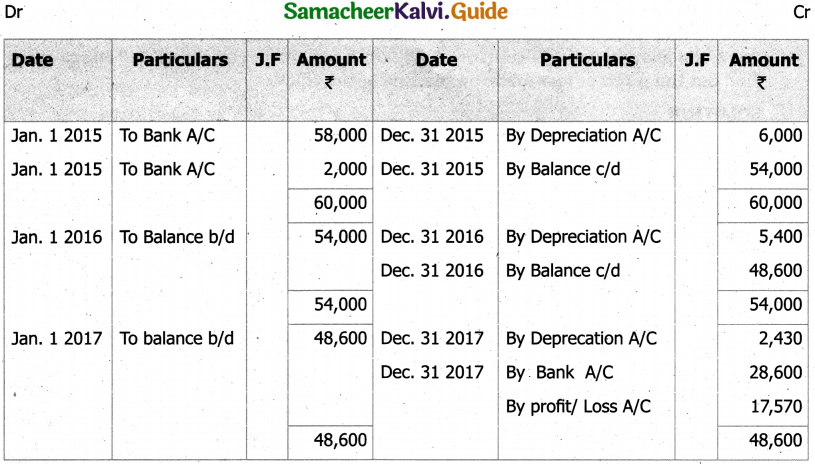Question 19.
Raj & Co purchased a machine on 1st January 2014 for ₹ 90,000. On 1st July 2014, they purchased another machine for ₹ 60,000. On 1st January 2015, they sold the machine purchased on 1st January 2014 for ₹ 40,000. It was decided that the machine be depreciated at 10% per annum on diminishing balance method. Accounts are closed on 31st December every year. Show the machinery account for the years 2014 and 2015.
Solution: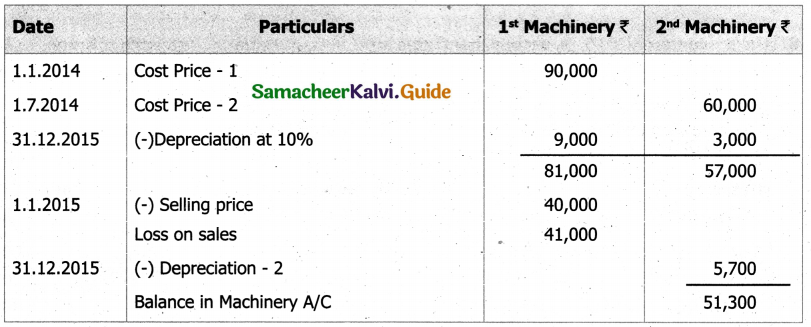Machinery Account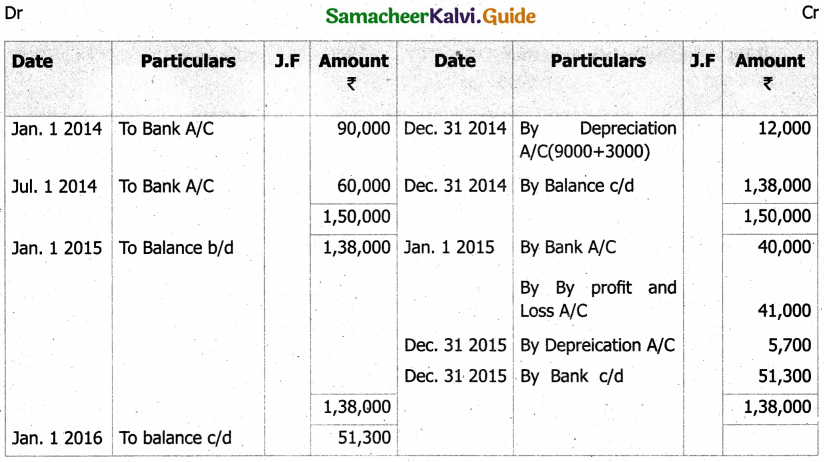Question 1.
Depreciation is calculated on ________ under diminishing balance method.
a) Original Cost
b) Written Down Value
c) The Scrap Value
d) None of the above
b) Written Down Value

Question 2.
Sinking Fund is also known as ________.
a) Depletion Method
b) Annuity method
c) Depreciation Fund method
d) None of the above
c) Depreciation Fund method

Question 3.
The process of becoming out of date or obsolete is termed as ________.
a) Depletion
b) Physical Deterioration
c) Obsolescence
d) None of the above
c) Obsolescence

Question 4.
In the process of provision method of depreciation the asset always valued at ________.
a) Market Price
b) Cost Price
c) Scrap Value
d) None
b) Cost PriceQuestion 5.
Meaning of Salvage value is ________.
a) Cash to be paid when asset is disposed off
b) Estimated disposal value
c) Definite sale price of the asset
d) Cash to be received when life of the asset ends
b) Estimated disposal value

Question 6.
In the accounting records, the fixed assets are normally recorded ________.
a) At Cost
b) At Book Value
c) At Scrap Value
d) At replacement value
a) At Cost

Question 7.
A fixed asset was bought for ₹ 5,000. Its accumulated depreciation is ₹ 1,000 and rate of depreciation is 10%. What are the depreciation expenses for the current accounting period using reducing balance method?
a) ₹ 600
b) ₹ 2000
c) ₹ 500
d) ₹ 400
c) ₹ 500

Question 8.
Under which depreciation method the amount of depreciation expenses remains same throughout the useful life of a fixed asset ________.
a) Straight Line Method
b) Reducing Balance Method
c) Number of Units produced method
d) Machine hour method
a) Straight Line Method

Question 9.
The book value of machinery on 01.04.2016 was ₹ 70, 000. Depreciation is charged at 10% p.a under Written Down value method on 31st March every year. The machine was sold for ₹ 50, 000 on 01.8.2017;calculate the Profit/Loss on sale of machinery.
a) Profit ₹ 5,755
b) Profit ₹ 5,000
c) Profit ₹ 10,375
d) Loss ₹ 10,325
c) Profit ₹ 10,375

Question 10.
The objectives of providing depreciation on an asset are ________.
a) To ascertain the true profit/loss of the firm
b) To provide funds for the replacement of the fixed assets
c) To show the true financial position of the firm
d) All of the above
d) All of the above

Question 11.
Cost of an asset is ₹ 3, 00,000. Rate of depreciation is 10% on WDV method. Value of the asset at the end of the second year will be ________.
a) ₹ 2, 70,000
b) ₹ 30, 000
c) ₹ 2, 50,000
d) ₹ 2, 43,000
d) ₹ 2, 43,000

Question 12.
Depletion method of charging depreciation is adopted for which of the following assets?
a) Plant and Machinery
b) Buildings
c) Wasting assets like mines and quarries
c) Wasting assets like mines and quarries

Question 13.
A trader followed WDV method of depreciation; the book value of assets after 4 years is 24% of original cost. Find rate of depreciation ________.
a) 24%
b) 26%
c) 32%
d) 30%
d) 30%

Question 14.
The older name of Straight line method is ________.
a) Annuity method
b) Revaluation method
c) Fixed Installment method
d) None
c) Fixed Installment methodQuestion 15.
Exhaustion is a ________ for depreciation.
a) Cause
b) Non-Cause
c) Both (a) & (b)
d) None
a) Cause

Question 16.
Under which method of depreciation, interest is also taken into consideration?
a) Revaluation method
b) Depletion method
c) Annuity method
d) None of the above
c) Annuity method

Question 17.
For oil wells ________ method of depreciation is to be followed.
a) Exhaustion
b) Wear & Tear
c) Depletion
d) None of the above
a) Exhaustion

Question 18.
Depreciation arises due to the following reason ________.
a) Wear & Tear
b) Fall in the market value
c) Effluxion of time
d) All the above
d) All the above

Question 19.
When the value of fixed assets increases it is known as ________.
a) Depreciation
b) Appreciation
c) Depletion
d) None
b) Appreciation

Question 20.
Depreciation on fixed assets is ________ expenditure.
a) Revenue Expenditure
b) Capital Expenditure
c) Deferred Revenue expenditure
d) None
b) Capital ExpenditureQuestion 1.
Define Depreciation.

1. According to Spicer and Pegler, “Depreciation is the measure of exhaustion of the effective life of an asset from any cause during a given period”.
2. According to R.N. Carter, “Depreciation is the gradual and permanent decrease in the value of an asset from any cause”.

Question 2.
What is ‘residual value’?

1. The amount which is expected to be realised at the end of the estimated useful life of an asset is known as scrap value of the asset. It is also known as residual value.
2. In determining the scrap value, costs to be incurred for removal and sale of the asset should be de-ducted from the estimated gross realisable value.

Question 3.
What is ‘Obsolescence’?

1. It is a reduction in the value of assets as a result of the availability of updated alternative assets.
2. This happens due to new inventions and innovations.
3. Though the original asset is in a usable condition, it is not preferred by the users and it loses its value.
4. For example, preference of latest computers by the users.

Question 4.
Write notes on ‘Effluxion of time’.
Certain assets whether used or not become potentially less useful with the passage of time.

Question 5.
What is ‘Straight Line Method’ of depreciation?

1. Under this method, a fixed percentage on the original cost of the asset is charged every year by way of depreciation. Hence it is called original cost method.
2. As the amount of depreciation remains equal in all years over the useful life of an asset it is also called as fixed instalment method.
3. When the amount of depreciation charged over its life is plotted on a graph and the points are joined together, the graph will show a horizontal straight line. Hence, it is called straight line method.

Question 6.
What is’Written down value’of depreciation?

1. Under this method, depreciation is charged at a fixed percentage on the written down value of the asset every year. Hence, it is called written down value method.
2. Written down value is the book value of the asset, i.e., original cost of the asset minus depreciation upto the previous accounting period.
3. As the amount of depreciation goes on decreasing year after year, it is called diminishing balance method or reducing installment method.

Question 7.
What is ‘Revaluation method’ of depreciation?

1. Under this method, the amount of annual depreciation is calculated by comparing the value of the assets at the end of the year and their value at the beginning of the year.
2. The value of the asset at the end of the year is determined with the consultation of relevant experts.
3. The excess of opening value over the closing value of the asset is the amount of depreciation for that year.
4. This method is used for live stock, loose tools, etc.Question 8.
What is ‘Insurance policy method’ of depreciation?

1. Under this method, an insurance policy is taken for an amount equal to the cost of replacement of the asset.
2. The amount of depreciation is paid by way of insurance premium every year to the insurance company.
3. On maturity of the policy, the policy amount is received from the insurance company and it is used for the purchase of new asset.

Question 9.
What are the factors determining the amount of depreciation?
i) Actual cost of the asset:

• Actual cost means the amount incurred in acquiring or constructing the asset.
• It is the acquisition or construction cost or historical cost. It includes all the expenses incurred on the asset to bring the asset to present condition and location, that is, all incidental expenses incurred till it is put into use.
• Purchase price of the asset, freight, loading charges, unloading charges, erection cost, setting up cost and expenses of trial run are included in the cost of the asset.
• If the asset is a second-hand one, the initial repair to make the asset useable is also to be taken as part of actual cost of the asset.

ii) Estimated useful life of the asset:

• The period for which an asset can be used in the enterprise is known as estimated useful life of an asset.
• It can be calculated in terms of period for which the asset is expected to be used by the entity or units of output to be obtained by the use of the asset, etc.
• In the case of intellectual properties like patents and copyrights, their legal life is taken as their estimated useful life.
• The Indian Companies Act, 2013 has prescribed useful lives of fixed assets for the purpose of com – putation of depreciation.
• For example, the useful lives prescribed in Part C of Section 123 for general plant and machinery and general furniture and fittings are 15 years and 10 years respectively.

iii) Scrap value of an asset:

• The amount which is expected to be realised at the end of the estimated useful life of an asset is known as scrap value of the asset. It is also known as residual value.
• In determining the scrap value, costs to be incurred for removal and sale of the asset should be de-ducted from the estimated gross realisable value.

iv) Other factors : Besides the above mentioned factors, legal provisions, technological factors, etc., also determine the amount of depreciation.

Question 10.
What are the Characteristics of depreciation?

1. Depreciation is the process of allocation of cost of depreciable asset (capital expenditure) to revenue expenditure or to profit and loss account over the useful life of the asset.
2. It is the process of allocation of cost and not the process of valuation.
3. It is a decrease in the book value of the asset and not the market value of the asset.
4. It is a gradual and continuous decrease in the book value of asset over its useful life.
5. It is calculated only for tangible depreciable fixed assets. Depreciation is not provided on intangible and wasting assets.

Question 11.
Find the amount of depreciation
Cost Price – ₹ 28,000 ; Estimated Life – 6 years; Scrap Value ₹ 4,000
Solution: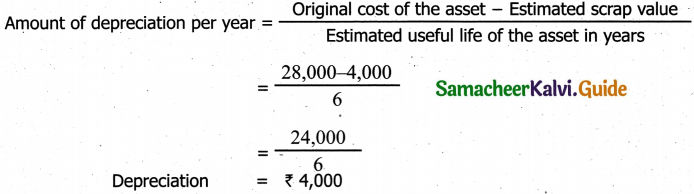Question 12.
On 1st January 2016, Anand Ltd., purchased a machine costing Rs.6, 000. It is estimated that its working life is four years and it will fetch no scrap value. The company decided to write off depreciation according to the fixed installment method. Prepare the machinery account.
Solution: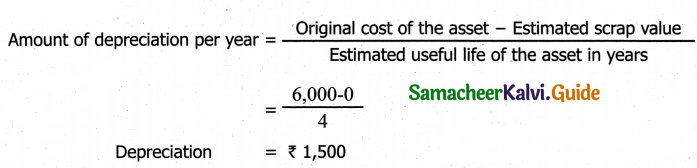Question 13.
A company purchased a plant for ₹ 2,00,000. The useful life of the asset is 10 years and the scrap value us ₹ 40, 000. Find the rate of depreciation under the straight line method.
Solution: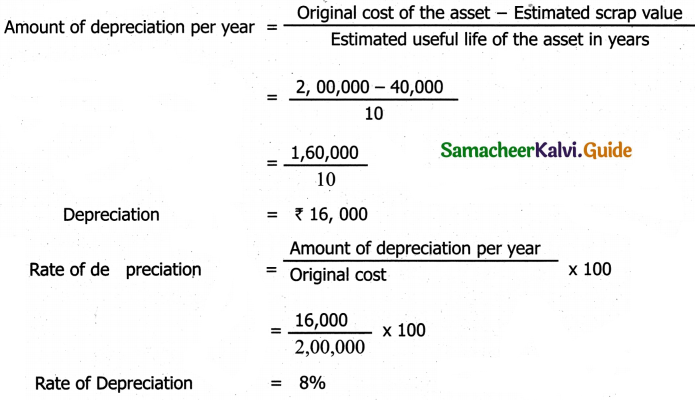Question 1.
Find out the rate of depreciation under straight line method.
Cost of asset – ₹ 10,000
Scrap value – ₹ 1,000
Estimated Life 10 years
Solution: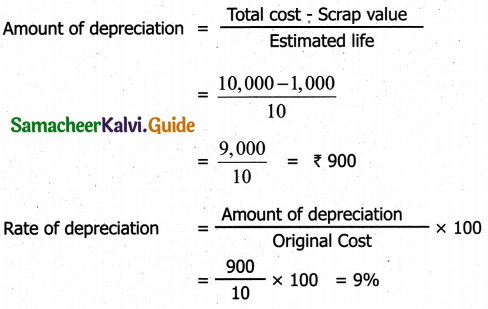Question 2.
Find out the rate of depreciation under straight line method.
Cost of Plants – ₹ 2,30,000
Installation charges – ₹ 20,000
Expected Life in year 10 years
Scrap value – ₹ 50,000
Solution: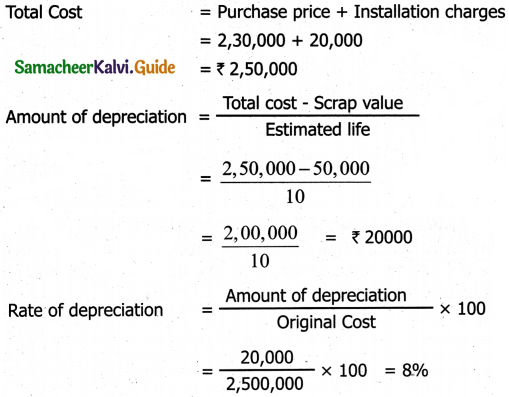Question 3.
A machine was purchased For ₹ 2,40,000, on 1.1.2010. This is expected to last for five year. Estimated scrap at the end of given year in ₹ 40,000. Find out the rate of depreciation under straight line method.
Solution: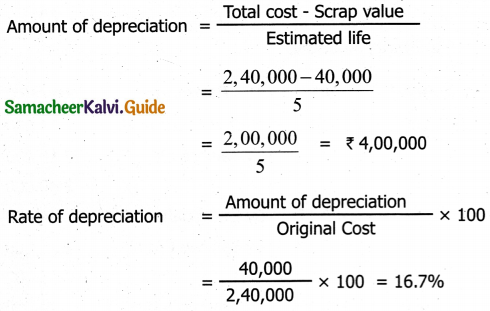Question 4.
A company has purchased a machinery for ₹ 1,70,000 and spent ₹ 20,000 for its installation. The estimated life of the machinery is 5 years with a residual value of ₹ 15,000. Find out the rate of depreciation under straight line method.
Solution: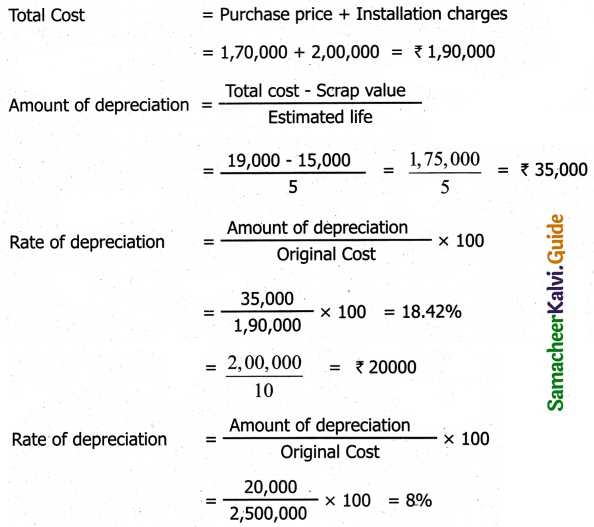Question 5.
Monisha Garments purchased a machinery on 1.4.2015 for ₹ 2,40,000. After three years the plane was sold for ₹ 1,80,000 The firm charges dpreciation at the rate of 10% per annum on stright line method. Accounts are closed on 31st march every year. Prepare machinery account and depreciation A/C.
Notes:
(i) Amount of Depreciation = ₹ 2,40,000
(ii) Book value after three year = 2,40,000 – (24000 x 3) = 2,40,000 – 72,000 = ₹ 1,68,000
(iii) Selling price = ₹ 1,80,000
Profit on sale of machinery = ₹ 72,000
Solution:
Machinary Account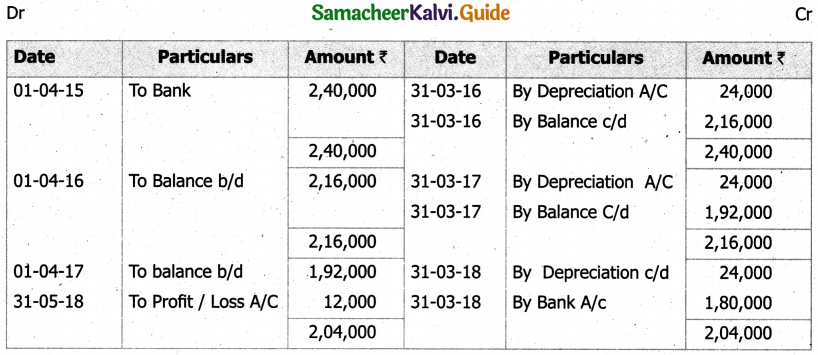Depreciation Account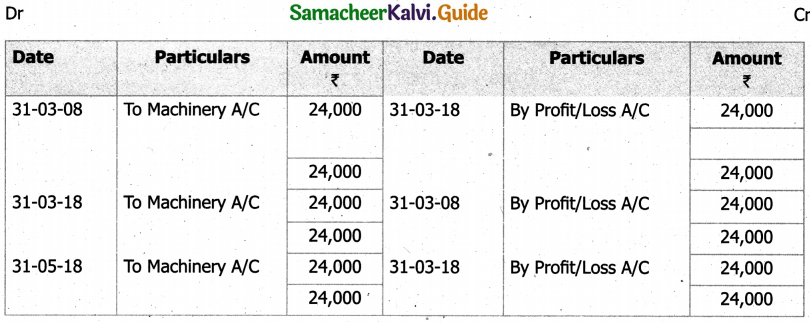Question 6.
Pugazh & CO, purchased a machinery for ₹ 4,70,000. On 1,4.2001 they spent₹ 30,000 on the repairs and installed the machinery. Depreciation is written after at 10% p.a on the straight Sine method on 31.3.2004, the machinery was found the unsuitable and sold for ₹ 3,50,000. Prepare machinery A/C assuming that the account are closed on 31st march every year.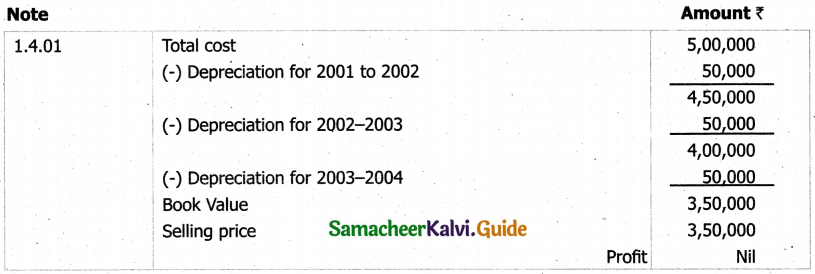Solution:
Machinery Account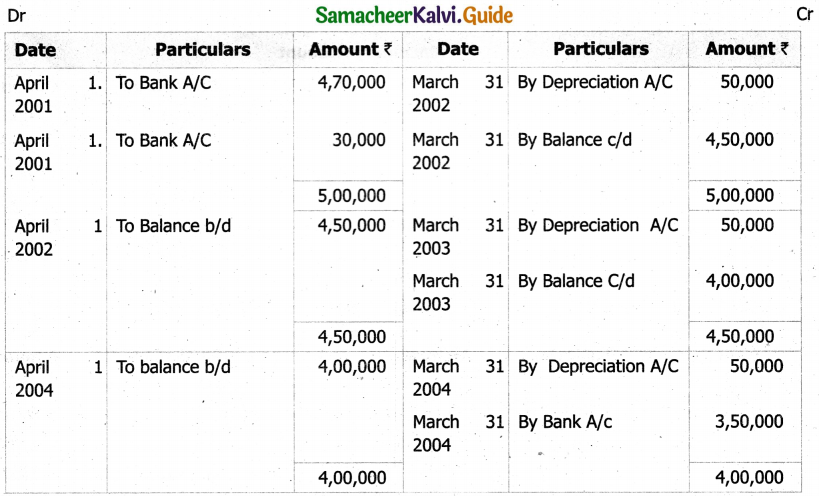Question 7.
A Company purchased a machinery on 1,4,2001 for ₹ 2,40,000 on 1 October 2002. It purchased another machinery for ₹ 60,000. On 1st October 2003, it sold oft the first machine purchased on 1.4.20C1 for ₹ 1,68,000 on the same date, it purchased another machinery for ₹ 1,50,000 Account are clsoed every year on 31st march depredation is written of at 10% p.a on original cost. Prepare machinery account and depreciation account for three years.
Solution:
I. Machinery purchased on 1.4.2001 for ₹ 2,40,000
1.4.2001 – 31.3.2002 = 24,000 – Ist year
1.4.2002- 31.3.2003 = 24,000 – IInd year
1.4.2003- 1.10.2003 = 12,000 – IIIrd year
Book value = 60,000
(-) Book value = 24,000 – 60,000 = ₹ 1, 80, 000
(-) Sale of machinery = ₹ 1, 68, 000
Loss on sale of machinery ₹ 12, 000

II. Machinery purchased on 1.10.2002 for Rs.60,000
1.10.2002 – 31.3.2003 = 3,000 – IInd year
1.4.2003 – 31.3.2004 = 6,000 – IIInd year

III. Machinery purchaed on 1.10.2003 for Rs.1,50,000
1.10.2003 – 31.3.2004 = 7,500 – IIIrd year

Machinary Account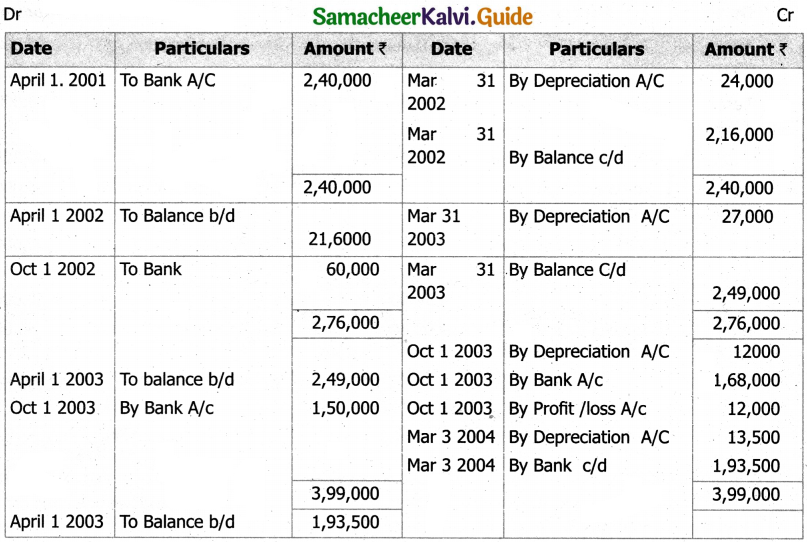Depreciation Account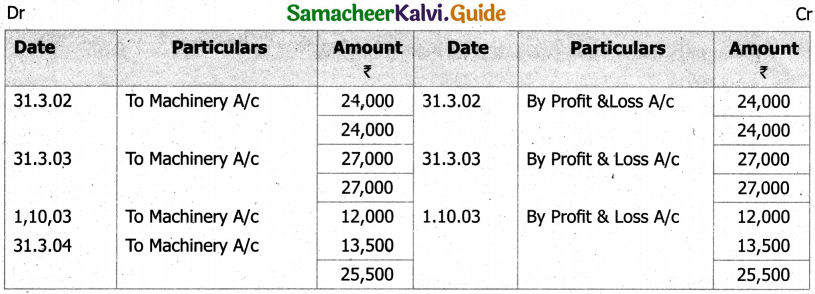Question 8.
A plant is purchased for ₹ 90,000, It is depreciation as 10% p.a on reducing balance for the three years. When it becomes obsolute due to new method of production and is scrapped. The scrap produces ₹ 66,000 at the end of the thrid year.
Prepare plant account for three year.
Solution:
Calculation of profit or loss on sales of plant.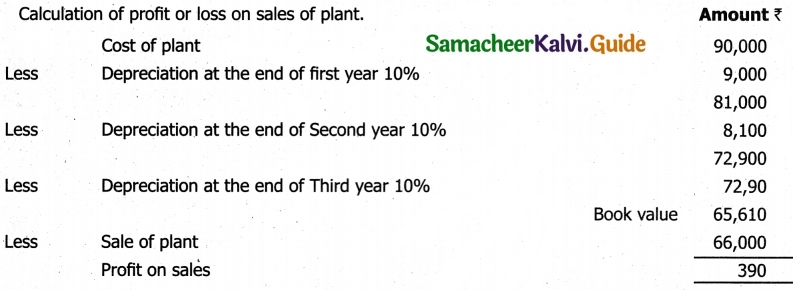Plant Account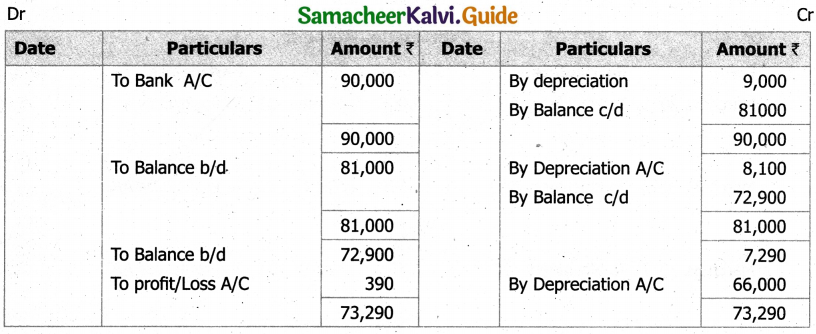Question 9.
A firm purchased a machine for ₹ 1,00,000 on 1-7-2015 depreciation is written oft at 10% on reducing balance method. The firm close its book on 31st December each year. Show the machinery account up to 31.12.2017
Solution:
Calculation of profit or loss on sales of machinery.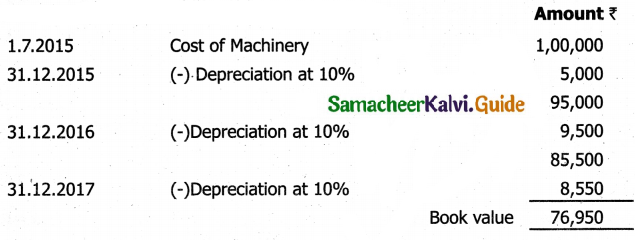Machinary Account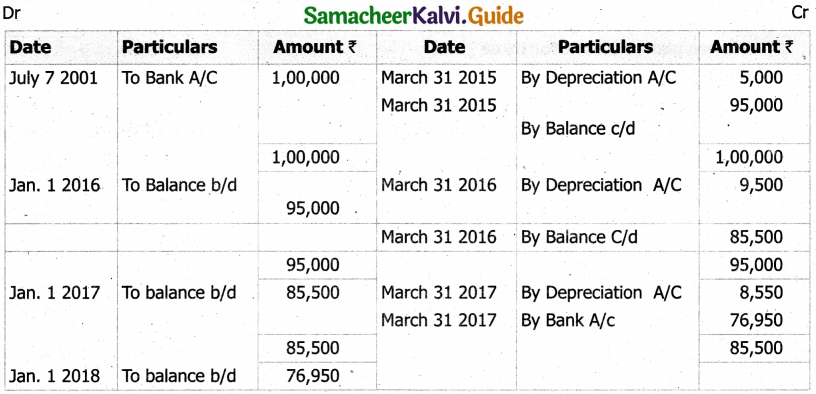Depreciation Account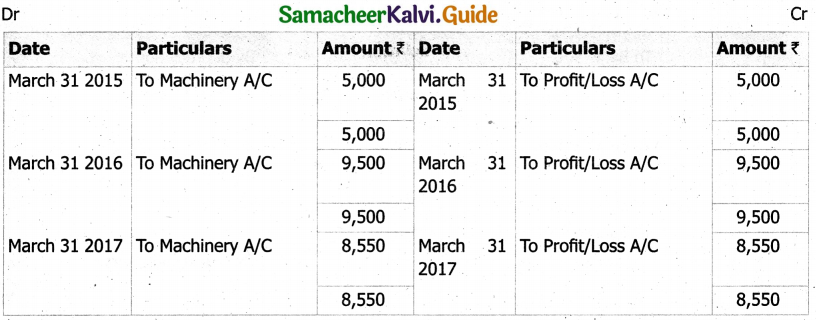Question 10.
Dhanuja shree started business on 1st April 2001 and she purchased a machinery for ₹ 1,40,000. She purchased another machinery on 1st November 2002 costing ₹ 30,000. She ( adopted a policy of charging 15% p.a depreciation under Diminishing balance method.
The account are closed every year on 31st march. Prepare machinery account and depreciation account for the first three years.
Solution:
Calculation of depreciation @ 15 % p.a.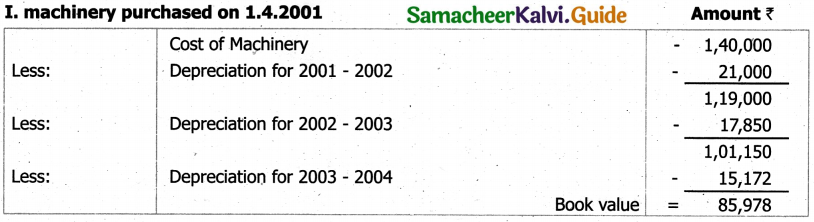Machinery Account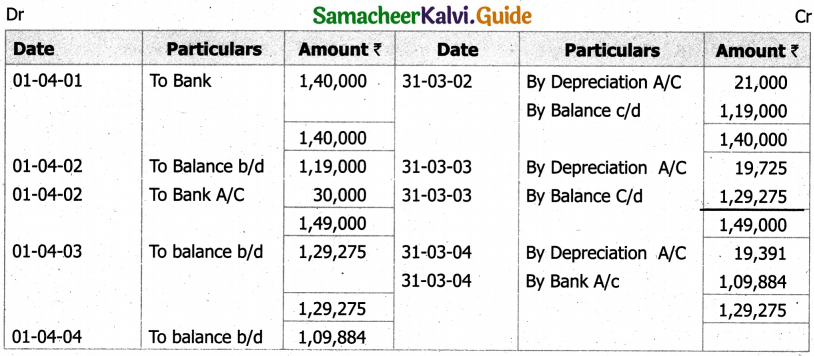Depreciation Account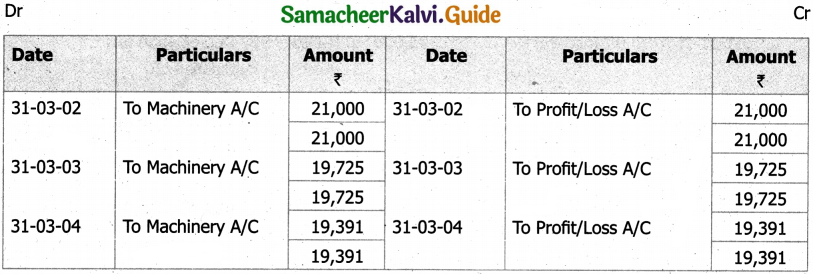Question 11.
On 1st January 2014, Hyagreeva Ltd., purchased a machine costing ₹ 12,000. It is estimated that its working life is four years and it will fetch no scrap value. The company decided to write off depreciation according to the fixed instalment method. Prepare the machinery account.
Solution: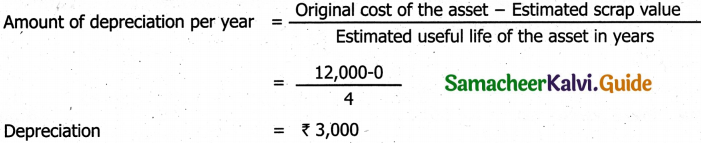Machinary Account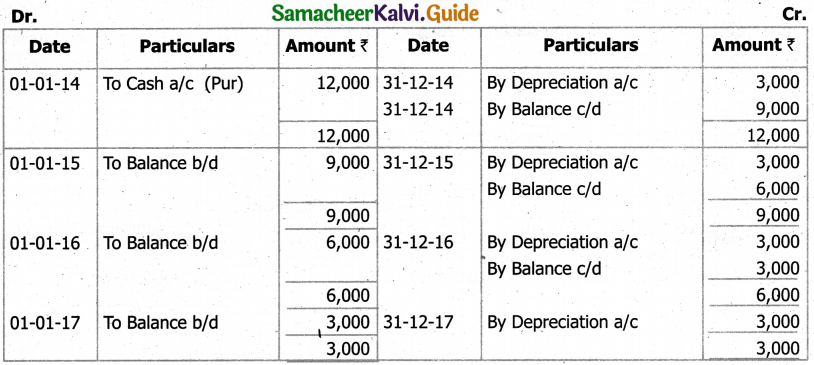Question 12.
Bharathi Ltd., purchased certain machinery at a cost of ₹ 40,000 on 1st January 2014. They decided to write off depreciation @ 20% p.a, according to straight line method. Prepare Machinery Account and Depreciation Account for the year 2014 to 2017.
Solution:
Machinary Account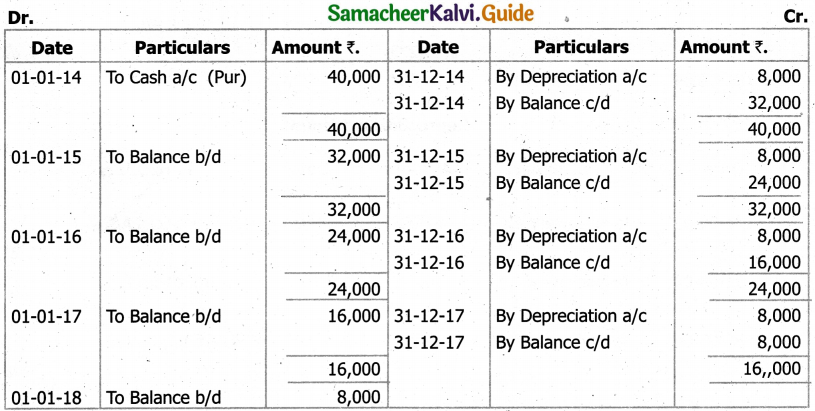Depreciation Account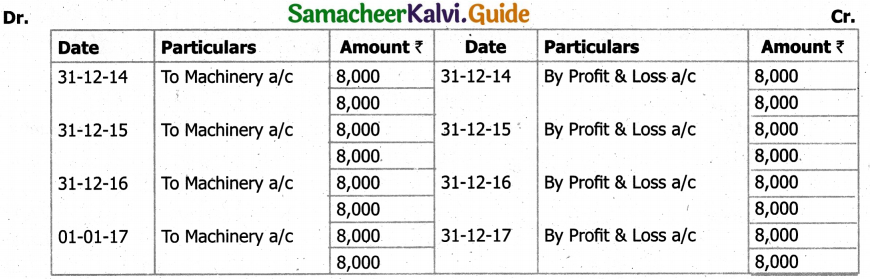Question 13.
Shreyan & Co., purchased a computer for ₹ 47,000 on 1st October 2012 and installed it by spending ₹ 3,000. Every year depreciations is to be charged at 10% on its cost. The computer is sold on IstJuly 2015 at a price of ₹ 35,000. Assuming that the accounts are dosed every year on December 31, prepare the computer account.
Solution:
Computer Account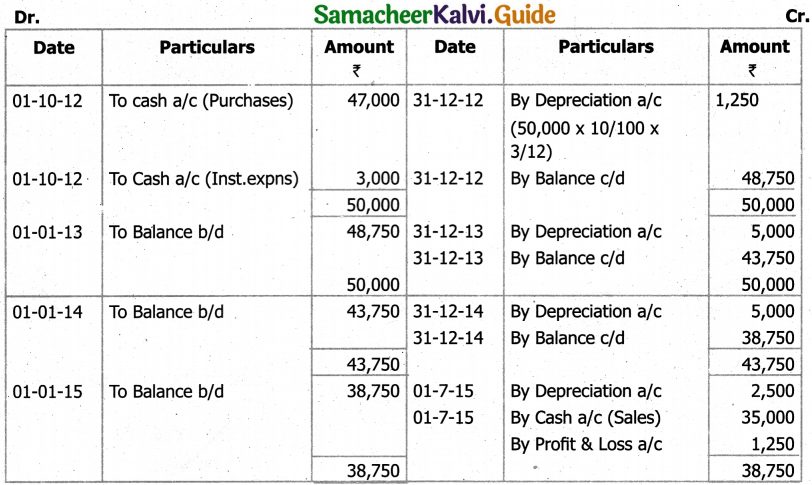Question 14.
A car was purchased on January 1, 2015 for Rs.80,000 depreciated at 10% on diminishing balance method. It was sold on 31st December 2017 for Rs.50,000. Prepare Car account.
Solution:
Car Account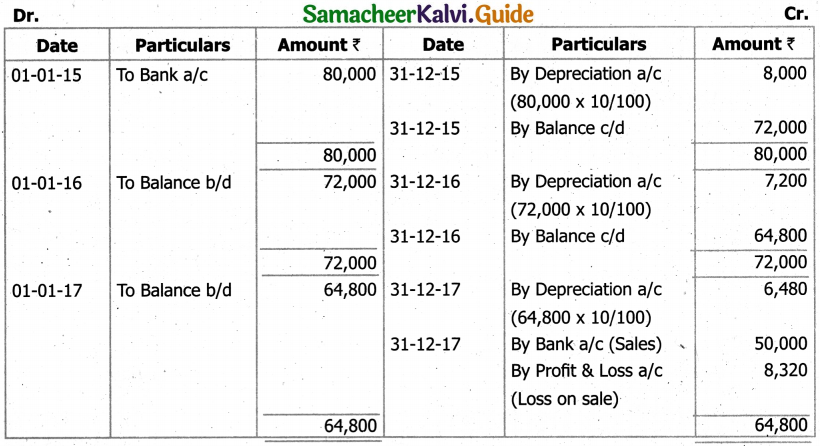Question 15.
In a business there was a machine for ₹ 90,000 on 1st January 2014. On 30.06.2014, another machinery was purchased for ₹ 10,000. On 31.12.2014 part of the machine was sold for 3,300, which had a cost price of ₹ 4,000 on 01.01.2014. Prepare machinery account after providing depreciation at 10% p.a on fixed installment basis.
Solution:
Machinary Account